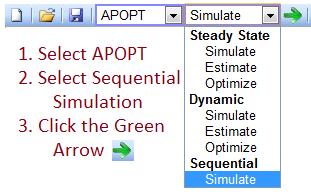## Van de Vusse Reactor

The Van de Vusse reaction kinetics are employed in many benchmarking problems. This model is a simple stirred tank reactor model with reactions A->B->C and 2A->D.Solve Van de Vusse Reactor Dynamics! Continuously Stirred Tank Reactor with energy
!   balance and Van de Vusse reactions A->B->C and 2A->D
!
! Optimization of Van de Vusse Reaction Kinetics Using Semibatch Reactor Operation
! G. A. Ridlehoover , R. C. Seagrave
! Ind. Eng. Chem. Fundamen., 1973, 12 (4), pp 444447
! DOI: 10.1021/i160048a008
! Publication Date: November 1973
!
Model
Parameters
F = 14.19         ! Feed rate (l/hr)
Qk = -1579.5      ! Jacket cooling rate (kJ/hr)
Ca0 =  5.1        ! Inlet feed concentration (mol/m^3)
T0 = 104.9        ! Inlet feed temperature (degC)

k10 = 1.287e10    ! A->B Pre-exponential factor (1/hr)
k20 = 1.287e10    ! B->C Pre-exponential factor (1/hr)
k30 = 9.043e9     ! 2A->D Pre-exponential factor (1/hr)
E1 = 9758.3       ! A->B Activation Energy (K)
E2 = 9758.3       ! B->C Activation Energy (K)
E3 = 8560         ! 2A->D Activation Energy (K)
dHr1 = 4.2        ! A->B Heat of Reaction (kJ/mol A)
dHr2 = -11        ! B->C Heat of Reaction (kJ/mol B)
dHr3 = -41.85     ! 2A->D Heat of Reaction (kJ/mol A)
rho = 0.9342      ! density (kg/l)
Cp = 3.01         ! Heat capacity of reactants (kJ/kg-K)
kw = 4032         ! Heat transfer coefficient (kJ/h-K-m^2)
AR = .215         ! Area of jacket cooling (m^2)
VR = 10.0         ! Reactor volume (l)
mK = 5            ! Mass of cooling (kg)
CpK = 2           ! Heat capacity of cooling (kJ/kg-K)
End Parameters

Variables
! Differential States
Ca = 2.2291       ! Concentration of A in CSTR (mol/l)
Cb = 1.0417       ! Concentration of B in CSTR (mol/l)
Cc = 0.91397      ! Concentration of C in CSTR (mol/l)
Cd = 0.91520      ! Concentration of D in CSTR (mol/l)

T  = 79.591  ! Temperature in CSTR (degC)
Tk = 77.69   ! Cooling jacket temperature (degC)
End Variables

Intermediates
k1 = k10*exp(-E1/(T+273.15))
k2 = k20*exp(-E2/(T+273.15))
k3 = k30*exp(-E3/(T+273.15))

r1 = k1*VR*Ca
r2 = k2*VR*Cb
r3 = k3*VR*Ca^2
End Intermediates

Equations
! note: the $denotes time differential ! (e.g.$x is dx/dt)

! species balances
VR * $Ca = -r1 - 2*r3 + F*(Ca0-Ca) VR *$Cb =  r1   - r2 - F*Cb
VR * $Cc = r2 - F*Cc VR *$Cd =  r3        - F*Cd

! energy balance on reactor
rho*Cp*VR*$T = F*rho*Cp*(T0 - T) & - r1*dHr1 & - r2*dHr2 & - r3*dHr3 & + kw*AR*(Tk - T) ! energy balance on cooling mK * CpK *$Tk = Qk + kw*AR*(T - Tk)
End Equations
End Model

File *.info
F, T0
F, F
F, Ca0
F, T0
F, Qk
S, Tk
S, Ca
S, Cb
S, Cc
S, Cd
C, T
End File

File overrides.dbs
nlc.web = 2
nlc.ctrl_units = 3
nlc.hist_units = 2
nlc.cv_type = 1
nlc.ev_type = 1
nlc.reqctrlmode = 1
nlc.csv_read = 1
nlc.hist_hor = 100
nlc.web_plot_freq = 10
nlc.nodes = 2
nlc.imode = 7
End File

File *.csv
time
0.0
0.1
0.2
0.3
0.4
0.5
0.6
0.7
0.8
0.9
1
1.1
1.2
1.3
1.4
1.5
1.6
1.7
1.8
1.9
2
2.1
2.2
2.3
2.4
2.5
2.6
2.7
2.8
2.9
3
End File